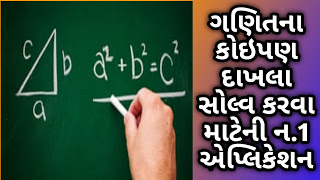# MY OJAS UPDATE

My Ojas Update :: Official Site Government Job, Private Jobs,Current Affairs, GK for Talati, TET,HTAT, Constable, Police,GPSC,UPSC,Results, Notifications.

Stay connected with MYOJASUPDATE for latest updates
Stay connected with MYOJASUPDATE for latest updates

## PHOTOMATH - CAMERA CALCULATOR ANDROID APPLICATION

Learn how to solve math problems, check homework assignments and study for upcoming exams and ACTs/SATs with the world’s most used math learning resource. Over 100+ million downloads, and billions of problems solved every month! Photomath is FREE and works without wi-fi.HOW IT WORKS
Instantly scan printed text AND handwritten math problems using your device’s camera or type and edit equations with our scientific calculator. Photomath breaks down every math problem into simple, easy-to-understand steps so you can really understand core concepts and can answer things confidently.

KEY FEATURES
• Scan textbook (print) AND handwritten problems
• Scientific calculator
• Step-by-step explanations for every solution
• Multiple solving methods
• No internet connection required to use
• 30+ languages supported
• Interactive graphs
MATH TOPICS
• Basic Math/Pre-Algebra: arithmetic, integers, fractions, decimal numbers, powers, roots, factors
• Algebra: linear equations/inequalities, quadratic equations, systems of equations, logarithms, functions, matrices, graphing, polynomials
• Trigonometry/Precalculus: identities, conic sections, vectors, matrices, complex numbers, sequences and series, logarithmic functions
• Calculus: limits, derivatives, integrals, curve sketching
• Statistics: combinations, factorials
Our in-house team of veteran math educators also partner with teachers worldwide to ensure we’re utilizing the most effective, contemporary teacher methodologies in our math engines.

Photomath is and will always be free to use, but you can boost your learning by upgrading to Photomath Plus. Photomath Plus provides solutions to full textbooks and problem sets with word problems! Currently restricted to the US and specific textbooks